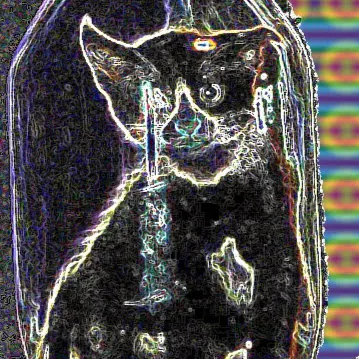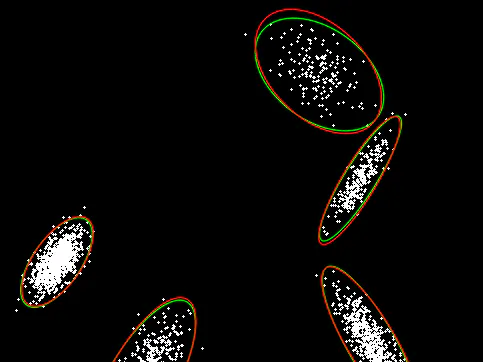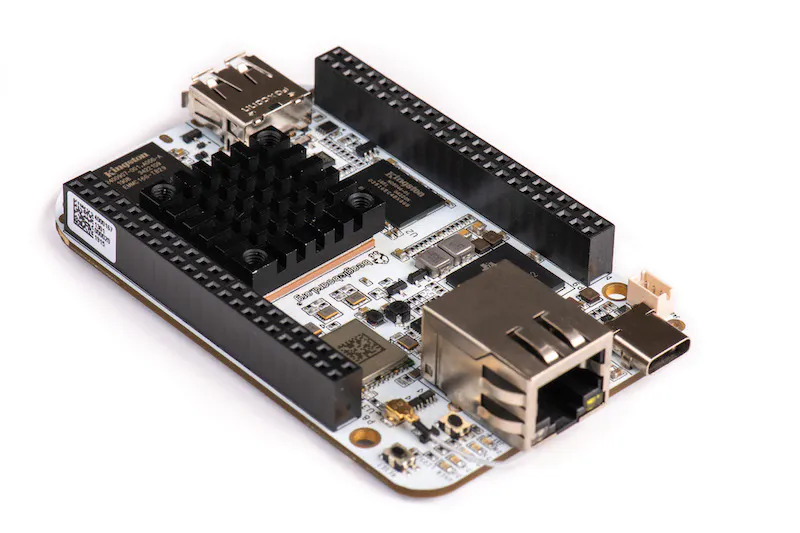Published

# Gaussian Distribution?

Hello... did you ever want to set up a gaussian distribution on your BeagleBoard. org family of boards, e.g. the BBAI?

BeginnerProtip1 hour2,741## Things used in this project

### Hardware componentsBeagleBoard.org BeagleBone AI
×1
 Fan 5v
×1
 USB 3.0 Hub
×1
 keyboard (USB)
×1
 mouse (USB)
×1
 Micro HDMI Cable to HDMI Cable
×1

 Debian

Here it is!

## Code

### Bell Curve (Circles)?

Python
This is it!
```#!/usr/bin/env python

# Python 2/3 compatibility
from __future__ import print_function
import sys
PY3 = sys.version_info == 3

if PY3:
xrange = range

import numpy as np
from numpy import random
import cv2

def make_gaussians(cluster_n, img_size):
points = []
ref_distrs = []
for i in xrange(cluster_n):
mean = (0.1 + 0.8*random.rand(2)) * img_size
a = (random.rand(2, 2)-0.5)*img_size*0.1
cov = np.dot(a.T, a) + img_size*0.05*np.eye(2)
n = 100 + random.randint(900)
pts = random.multivariate_normal(mean, cov, n)
points.append( pts )
ref_distrs.append( (mean, cov) )
points = np.float32( np.vstack(points) )
return points, ref_distrs

def draw_gaussain(img, mean, cov, color):
x, y = np.int32(mean)
w, u, vt = cv2.SVDecomp(cov)
ang = np.arctan2(u[1, 0], u[0, 0])*(180/np.pi)
s1, s2 = np.sqrt(w)*3.0
cv2.ellipse(img, (x, y), (s1, s2), ang, 0, 360, color, 1, cv2.LINE_AA)

if __name__ == '__main__':
cluster_n = 5
img_size = 512

print('press any key to update distributions, ESC - exit\n')

while True:
print('sampling distributions...')
points, ref_distrs = make_gaussians(cluster_n, img_size)

print('EM (opencv) ...')
em = cv2.ml.EM_create()
em.setClustersNumber(cluster_n)
em.setCovarianceMatrixType(cv2.ml.EM_COV_MAT_GENERIC)
em.trainEM(points)
means = em.getMeans()
covs = em.getCovs()  # Known bug: https://github.com/opencv/opencv/pull/4232
found_distrs = zip(means, covs)

img = np.zeros((img_size, img_size, 3), np.uint8)
for x, y in np.int32(points):
cv2.circle(img, (x, y), 1, (255, 255, 255), -1)
for m, cov in ref_distrs:
draw_gaussain(img, m, cov, (0, 255, 0))
for m, cov in found_distrs:
draw_gaussain(img, m, cov, (0, 0, 255))

cv2.imshow('gaussian mixture', img)
ch = cv2.waitKey(0)
if ch == 27:
break
cv2.destroyAllWindows()
```

## Credits

### Seth

29 projects • 11 followers
Stay there and someone will find you...
Thanks to Beagleboard.org people for Producing the AI, OpenCV developers, and Unknown for now.